﻿ Technical Notes > Applications in Electromagnetics > Waveguides > Homogeneous Waveguides

Homogeneous Waveguides

Navigation:  Technical Notes > Applications in Electromagnetics > Waveguides >

Homogeneous WaveguidesIn many cases, the waveguide under analysis consists of a metal casing, either empty or filled homogeneously with an isotropic dielectric.  In these cases, the analysis can be simplified.

Eq. (3.3) can be expanded using (3.5) and rearranged to express the transverse x and y components in terms of the axial z components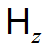and.
(3.6)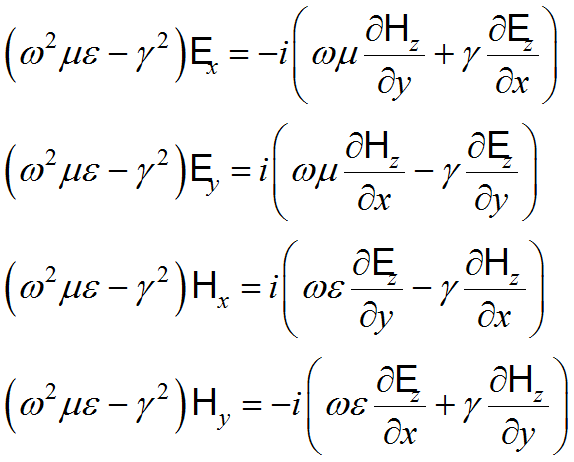The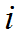in the right hand side corresponds to a phase shift of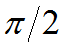in the expansion (3.1).

Applying, the divergence equations of (3.3) become
(3.7)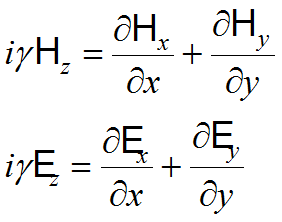,

so  the z component equations of (3.4) are
(3.8)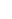.These are eigenvalue equations in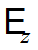and, and the values offor which solutions exist constitute the propagation constants of the unattenuated propagation modes that can be supported in the guide under analysis.  For any eigenvalue, there are an infinite number of combinations ofwhich can excite this mode, and the exact determination will depend on the materials and the driving frequency.